# Conversion of Flip Flops

Conversion of flip-flops causes one type of flip-flop to behave like another type of flip-flop. In order to make one flip-flop mimic the behavior of another certain additional circuitry and/or connections become necessary.

### Conversion of JK Flip-Flop to SR Flip-Flop

Step 1: Write the Truth Table of the Desired Flip-Flop
Here SR flip-flop is to be designed using JK flip-flop. Thus one needs to write the truth table for SR flip-flop.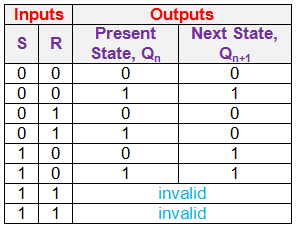Step 2: Obtain the Excitation Table for the given Flip-Flop from its Truth Table
Excitation tables provide the details regarding the inputs which must be provided to the flip-flop to obtain a definite next state (Qn+1) from the known current state (Qn).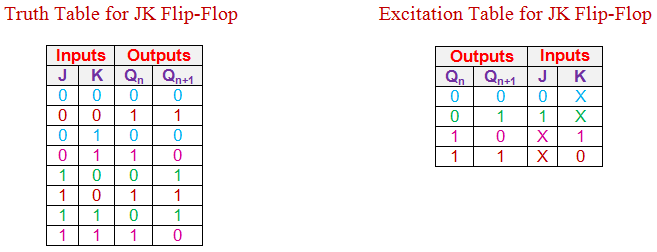From the truth table of JK flip-flop one can see that Qn+1 will become 0 from Qn = 0 for both (i) J = K = 0 and (ii) J = 0 and K =1 (blue entries in first and third rows of the truth table).

This means that to obtain the next state, Qn+1 as 0 from the current state Qn = 0, J must be made zero while K can be either 0 or 1.

This is indicated by the first row of the excitation table (blue entries in the first row of excitation table) where the value of K is expressed as ‘X’ indicating don’t care condition. Similarly to obtain the next state as 1 from the current state 0, one has to have J equal to 1 while K can be either 0 or 1 (indicated by green entries of the truth table).

This leads to the second row of excitation table (green entries) to be filled with values Qn = 0, Qn+1 = 1, J = 1 and K = X. On the same grounds, the entire excitation table needs to be filled (entries in pink and dark red colors).

Step 3: Append the Excitation Table of the given Flip-Flop to the Truth Table of the Desired Flip-Flop Appropriately to obtain Conversion Table
Here the conversion table is obtained by filling-up the values of the J and K inputs for the given Qn and Qn+1, by referring to the excitation table.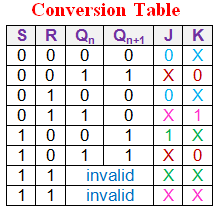Step 4: Simplify the Expressions for the Inputs of the given Flip-Flop
In this case, one needs to arrive at the logical expressions for the inputs J and K in terms of S, R, and Qn using suitable simplification techniques like K-map.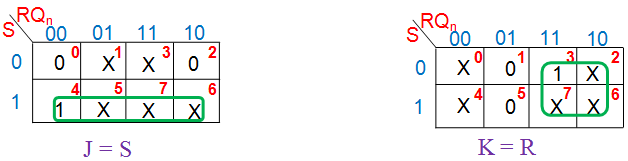Step 5: Design the Necessary Circuit and make the Connections accordingly
Here neither additional circuit nor new connections are necessary.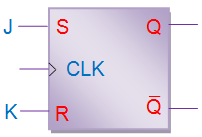On the same grounds, one can convert the given flip-flop to any other type of flip-flop as shown below.

### Conversion of JK Flip Flop to D Flip Flop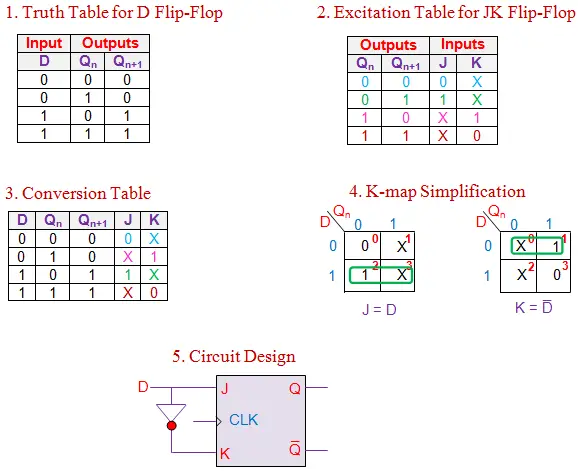### Conversion of JK Flip Flop to T Flip Flop

The conversion from a JK flip flop to a T flip flop is shown below.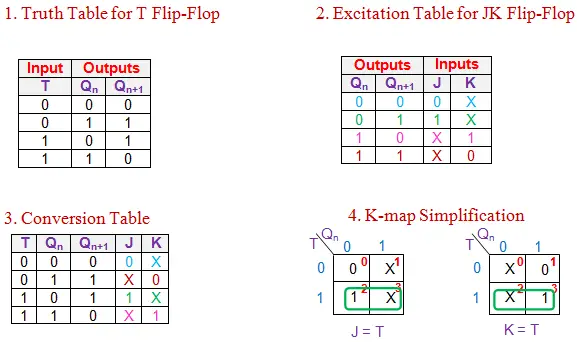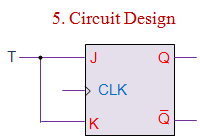### Conversion of SR Flip Flop to JK Flip Flop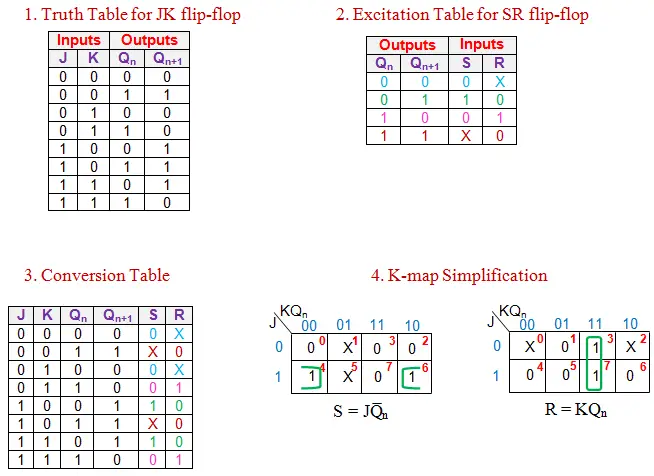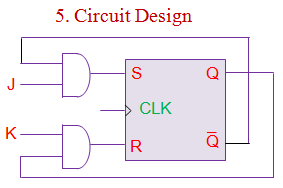### Conversion of SR Flip Flop to D Flip Flop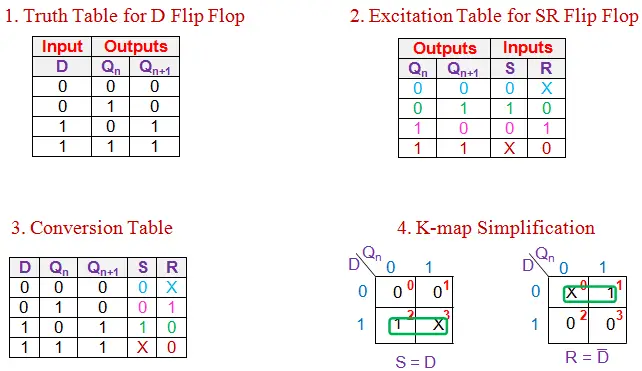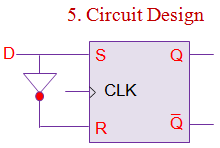### Conversion of SR Flip Flop to T Flip Flop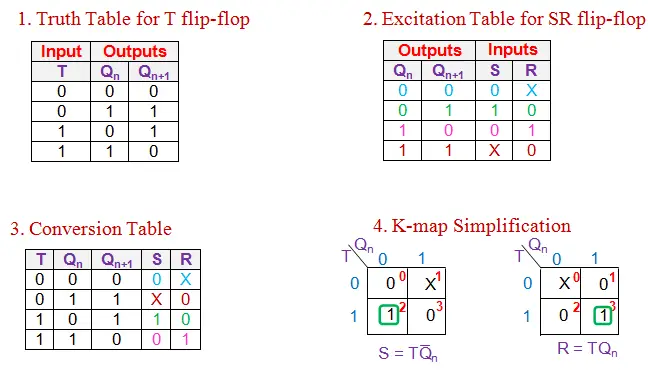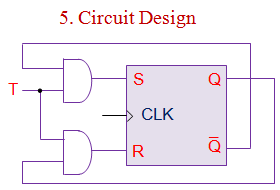### Conversion of D Flip Flop to JK Flip Flop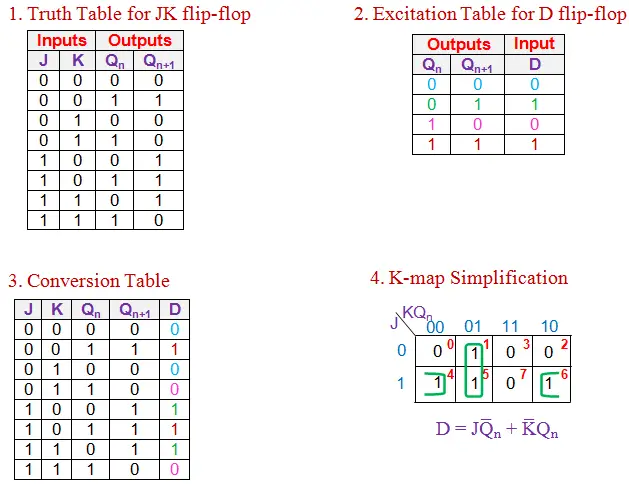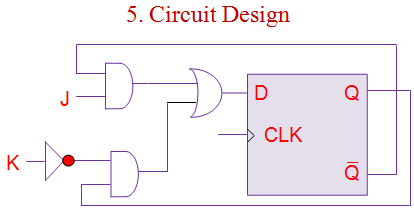### Conversion of D Flip Flop to SR Flip Flop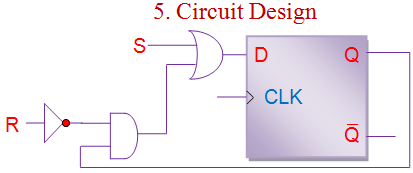### Conversion of D Flip Flop to T Flip Flop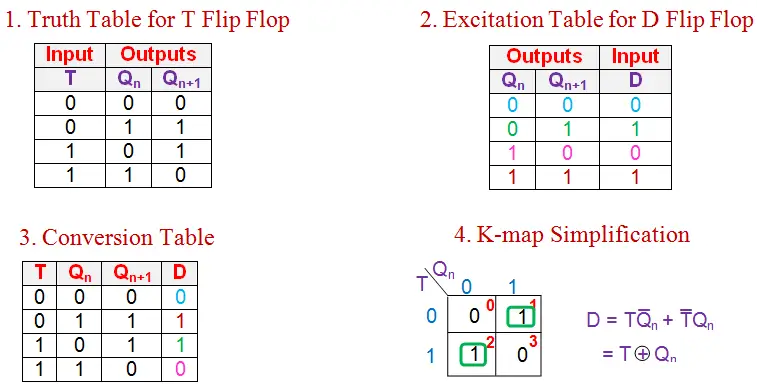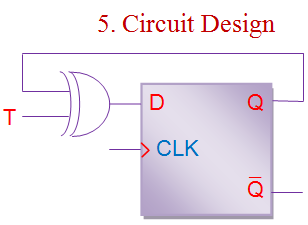### Conversion of T Flip Flop to JK Flip Flop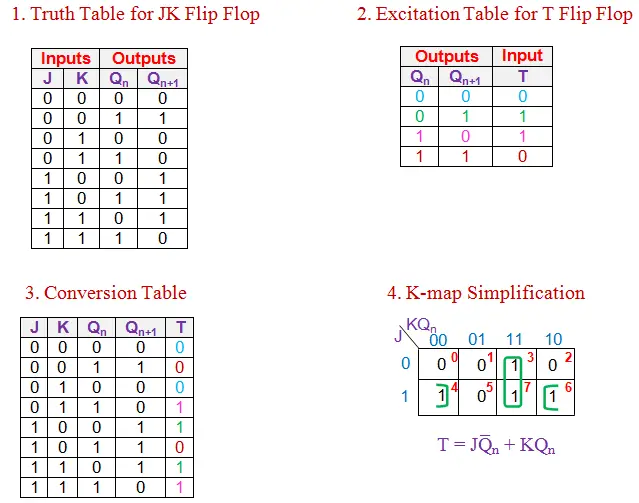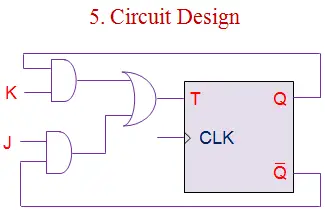### Conversion of T Flip Flop to SR Flip Flop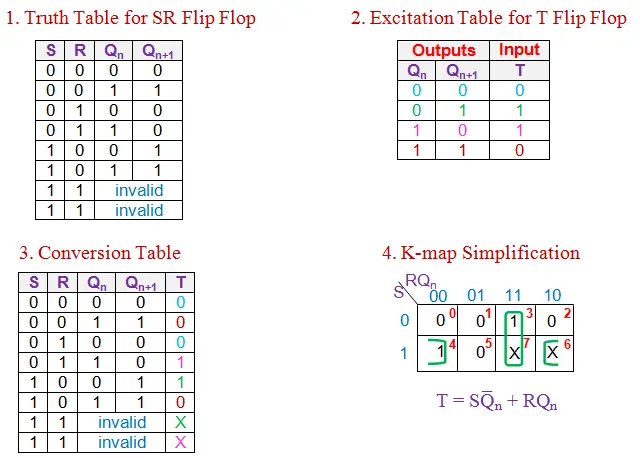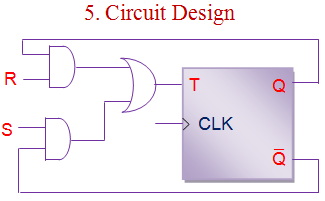### Conversion of T Flip Flop to D Flip Flop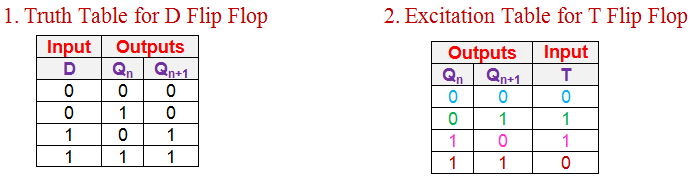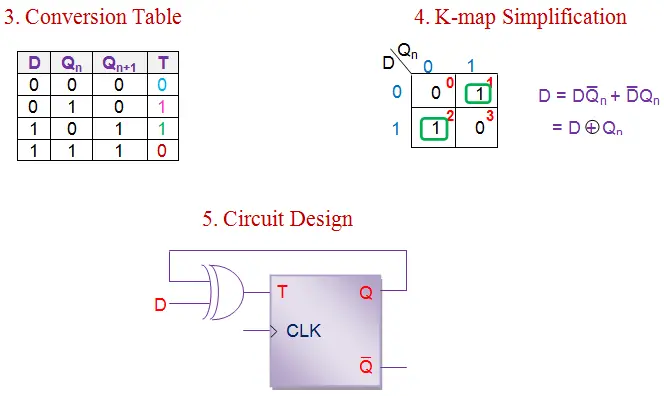Want To Learn Faster? 🎓
Get electrical articles delivered to your inbox every week.
No credit card required—it’s 100% free.